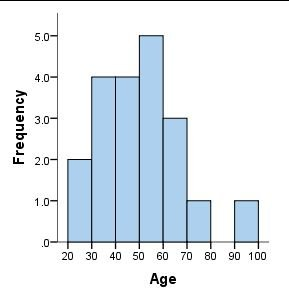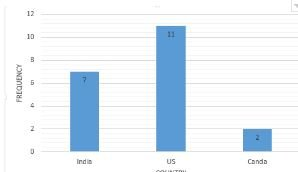# What is the difference between Histogram & Bar Chart?

Standard
 Histogram Bar ChartThe x-axis represents bins. So if you have a continuous variable like age which has values from 0-100 then you can create bins like 0-10, 10-20 and so on (and here bin size = 10). You can change the bin size to analyze the distribution of the data. X-axis has a numerical (quantitative) variable. The x-axis represents distinct categories from your data. The variable on the x-axis is usually qualitative The order of the bins is important since it is used to understand the distribution of the data. The order of the categories in the bar chart doesn’t matter. We can sort it if we want but it’s not needed.

# Hadoop on Azure’s Javascript Interactive Console has basic graphing functions:

Standard

The Hadoop on Azure’s Javascript console has basic graphing functions: Bar, Line & Chart. I think this is great becuase it gives an opportunity to visualize data that’s in HDFS directly from the Interactive Javascript Console! Here’s a screenshot:In the console, I ran the help(“graph”) command to see how I can use this function:
Draw a graph of data
graph.bar(data, options) Bar graph
graph.line(data, options) Line graph
graph.pie(data, options) Pie chart

Parameters
data (array) Array of data objects
options (object) Options object, with
x (string) Property to use for x-axis values
y (string) Property to use for y-axis values
title (string) Graph title
orientation (number) x-axis label orientation in degrees
tickInterval (number) x-axis tick interval

##### Conclusion:

In this blog-post, I posted that Hadoop on Azure’s Javascript Interactive Console has basic graphing functions.